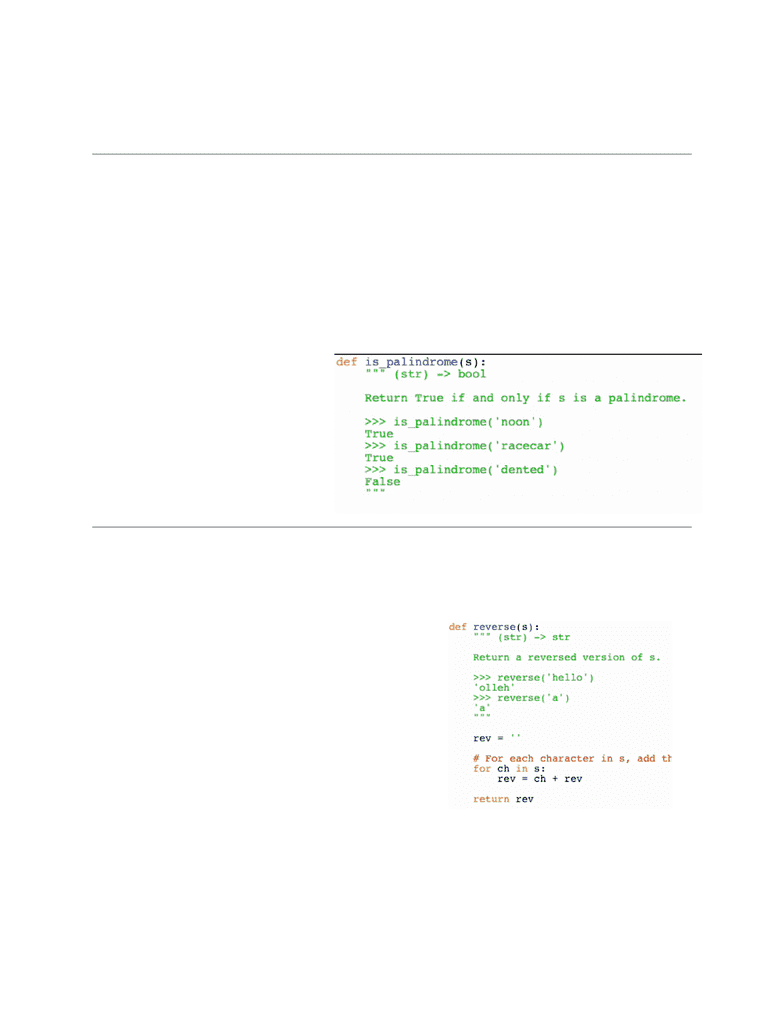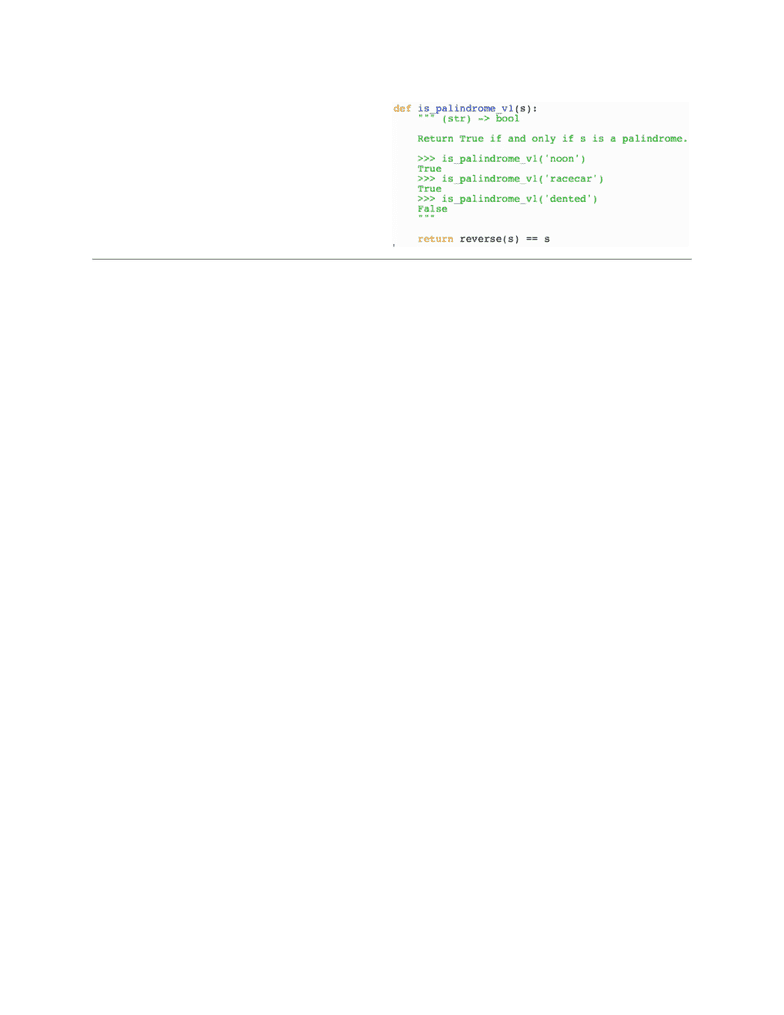Class Notes (1,100,000)
CA (620,000)
UTSC (30,000)
All (10)
Lecture 8

# CSCA08 Lecture 8: Week 8 Notes

Department
Computer Science
Course Code
CSCA08H3
Professor
All
Lecture
8

This preview shows pages 1-2. to view the full 7 pages of the document.Alexander Magony
CSC108H1F 2014
Week 8
Palindrome: Approaching the Problem
-Problem solving: determining whether a string is a palindrome (read the same way in either
direction like ‘racecar or ‘noon’).
Algorithm 1: if the string is a palindrome, the reversed string is the same as the original so
we can compare them.
Algorithm 2: can split the string into two halves (‘noon’ into ‘no on’ and reverse the
second half to get ‘no no’). If both halves are equivalent, it is a palindrome. If an odd
number of character, you ignore the middle character (eg. ‘e’ in ‘racecar’).
Algorithm 3: can compare pairs of characters (last and ﬁrst character, second and second
last character, etc). If all the same, it is a palindrome. Stop when the middle is reached.
Again, if odd, you can ignore
the middle character.
The examples, type contract,
header, and description were
created (in that order) as
shown to the right.
NOTE: the body and testing
will be done in subsequent
parts.
Palindrome: Algorithm 1
-Reminder: algorithm 1 uses the approach of comparing the reversed string to the original
string to establish if it is a palindrome.
-Unlike lists, there is no reverse method or function
for strings so we must create one.
This function must reverse, for example, ‘hello’ to
‘olleh’ or ‘a’ to ‘a’.
we need an accumulator rev to build the new
reversed string.
Since we are going through the characters of the
original string in order but must add it to the
accumulator in a reversed fashion. (eg. rev = ch +
rev rather than rev = rev + ch) This adds each
subsequent character to the beginning of the
accumulator string so we build a reversed version
of the original string.
After this string is built, we can simply return it.
-Now we can create the function that checks if a string is a palindrome. We simply have to
compare the string given to a reversed version of the string (using our newly created reverse
function).
1

Only pages 1-2 are available for preview. Some parts have been intentionally blurred.Alexander Magony
CSC108H1F 2014
-The ﬁnished function is shown to the
right (the body really just says return
reverse(s) == s since the reverse
function did the hard part.
Palindrome: Algorithm 2
-Reminder: algorithm 2 uses the approach of splitting the string into two halves and comparing
a reversed second half to the ﬁrst half.
Again, we need a reversed version of a string, so we can reuse our reverse(s) function.
Also remember that we cannot use the middle character of a string with an odd number of
characters (since we cannot split this middle character in half). Eg. in racecar’s case, we
would only take car and reverse it to compare with rac.
We need a way of ﬁnding the starting index of the second half so we can slice it. len(s) -
len(s)/2 seems to work.
-With racecar, an odd-numbered string, the length is 7 and the integer division by 2 is 3. 3
is the index of the middle character so we can’t use that. When we subtract 7-3, we get
4, the index of the ﬁrst letter after the middle character e. This works.
-With noon, an even-numbered string, the length is 4 and the integer division is 2. 4-2 is
2, which is the index of the third character in the string which is the ﬁrst index of the
second half. This works.
-Generally, it seems len(s) // 2 gives us the length of each half/slice. Since we want the
start of the second half, we need to count the length of that slice back from the end of
the full string. This is why we subtract the length of one slice of the string from the full
string.
-To actually build the body of the function now:
We know that we want to grab the ﬁrst half. The length of one half is the length of the full
string integer-divided by 2. So to actually slice the ﬁrst half, we can slice from the beginning
of the string to the index of the length of a half. s[ : len(s) // 2]
-In the case of racecar, one half is 3 characters. Index 3 is e, so slicing s[ : 3] would slice
everything up to but not including e, which is what we want.
We want to compare this to a reversed version of the second half slice. We’ve said that the
starting index is the length of the full string minus the length of a half slice and we want to
slice everything from that point until the end of the string. s[ len(s) - len(s) // 2 : ].
-In the case of racecar, 7-3 is 4. The 4th index is c which is what we want the start index
to be.
Of course, we want to check if the ﬁrst half is compared to a reversed second half (using
our reverse function). So the body should contain the boolean comparison: return s[ :
len(s) // 2] == s[len(s) - len(s) // 2: ].
-This can be a bit convoluted, so we may choose to ﬁrst make a variable n = len(s) so we
can simplify the above statement. So we get return s[ : n // 2 ] == s[n - n // 2: ]
The completed function is shown below.
2
###### You're Reading a Preview

Unlock to view full version